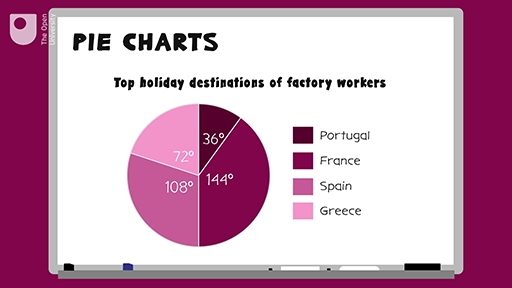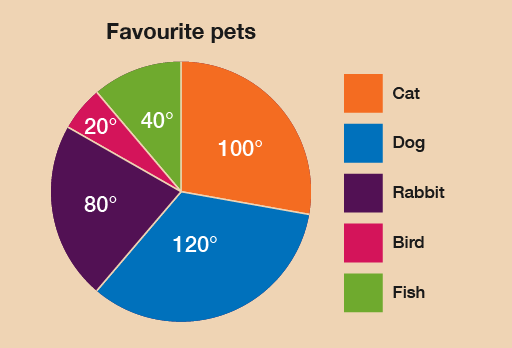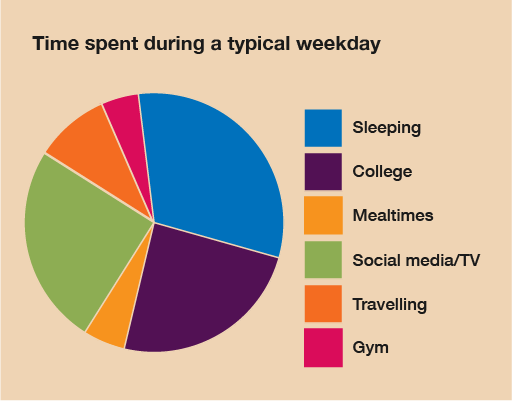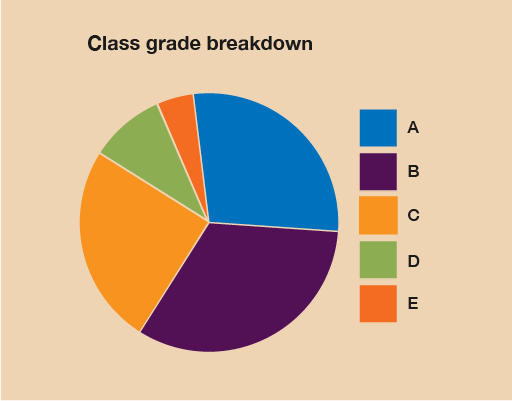Science, Maths & Technology

### Become an OU studentEveryday maths 1 (Wales)

Start this free course now. Just create an account and sign in. Enrol and complete the course for a free statement of participation or digital badge if available.

# 4 Pie charts

Charts are basically maths pictures. There are two types of charts: bar charts, which you’ll look at in the next section, and pie charts.

Pie charts are a clear way of presenting data, but they can be difficult to draw and the calculations involved in creating them can be complicated.

A pie chart is a circle (or ‘pie’) divided in sections (or ‘slices’). The sizes of these sections represent the data. Pie charts must contain both a title and a key that explains what each section means.

## Example: Soap operas

How would you present information as a pie chart? Watch the following video to find out.Interactive feature not available in single page view (see it in standard view).

### Method summary

• Find out what the whole of the ‘pie’ is going to represent – this is the total of your categories added together.
• Divide 360 (the size of a circle in degrees) by this total to tell you what one unit of your data makes. Use a calculator if you need to!
• Multiply the amount for this one unit by the size of each category.
• This gives the size of what each segment should be (in degrees).
• Draw a circle and draw a line from the middle of the circle to the top.
• Starting from this line, use a protractor to measure and draw each slice.
• Label the slices, or use a key to show what each segment represents.
• Give your pie chart a title.

Now try the following activity. If you get stuck, refer to the method summary above, and remember to check your answers once you have completed the questions.

## Activity 10: Creating a pie chart

Calculate the answers to the following problems without using a calculator. You may double-check your answers with a calculator if you need to. Remember to check your answers once you have completed the questions.

In a survey, 18 people were asked what their favourite pets were. The responses were as follows:

Pet Number of people
Cat 5
Dog 6
Rabbit 4
Bird 1
Fish 2

Draw a pie chart to represent this information.

To find out how many degrees each animal is represented by, you must carry out this calculation:

• 360 ÷ 18 = 20

Therefore, each animal is represented by 20°. We can then calculate the size of each section:

Pet Number of people Angle
Cat 5 5 × 20° = 100°
Dog 6 6 × 20° = 120°
Rabbit 4 4 × 20° = 80°
Bird 1 1 × 20° = 20°
Fish 2 2 × 20° = 40°

As a check, you can make sure that the degrees for each section add up to 360° in total:

100° + 120° + 80° + 20° + 40° = 360°

From these measurements you should construct a pie chart as follows:Figure 10 Pets pie chart

Bill records how he spends his time over a typical day. Draw a pie chart to represent this information:

Activity Time spent
Sleeping 8 hours
College 7 hours
Eating 1 hour
Social media 5 hours
Travelling 2 hours
Gym 1 hour

The time spent on activities adds up to 24 hours – as you would expect! To find out how many degrees each hour is represented by, you must carry out this calculation:

360 ÷ 24 = 15

Therefore, each hour is represented by 15°. We can then calculate the size of each section:

Activity Time spent Angle
Sleeping 8 hours 8 × 15 = 120
College 7 hours 7 × 15 = 105
Eating 1 hour 1 × 15 = 15
Social media 5 hours 5 × 15 = 75
Travelling 2 hours 2 × 15 = 30
Gym 1 hour 1 × 15 = 15

You can check these figures by making sure that the degrees for each section add up to 360° in total:

120° + 105° + 15° + 75° + 30° + 15 = 360°

Using these values you can now draw your pie chart – don’t forget to add a title and a key. From these measurements your pie chart should look like this:Figure 11 Daytime activities pie chart

A tutor records the test results for a class:

A 10
B 13
C 7
D 4
E 2

Draw a pie chart to show the breakdown of results.

The total number of students is 36. To find out how many degrees each student is represented by, you must carry out this calculation:

360 ÷ 36 = 10

Therefore, each hour is represented by 10°. We can then calculate the size of each section:

A 10 10 × 10 = 100
B 13 13 × 10 = 130
C 7 7 × 10 = 70
D 4 4 × 10 = 40
E 2 2 × 10 = 20

Using these values you can now draw your pie chart, which should look like this:Figure 12 Grade breakdown pie chart

## Summary

In this section you have learned about how to present data in pie charts.